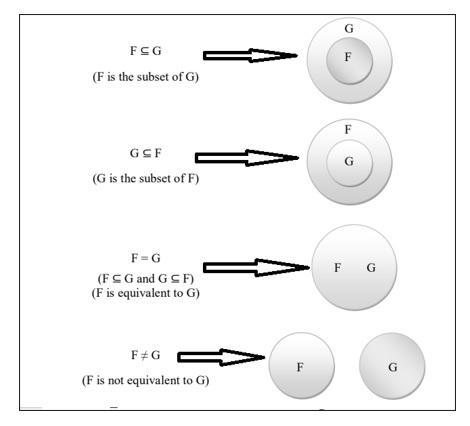# What is an equivalence of sets of functional dependencies?

A set of functional dependencies (FD) F is said to cover another set of functional dependencies E if every FD in E is also in F closure; that is, if every dependency in E can be inferred from F.

Alternatively, we can say E is covered by F. Two sets of functional dependencies E and F are equivalent if E+= F+. That is E is equivalent to F if E covers F and F covers E.

To determine whether F covers E we calculate X+ with respect to F for each FD X->y in E and then check whether X+ includes the attributes Y.

## Example

R=(A,B,C,D,E,F)

F1={A->BC, B->CDE, AE->F}

F2={A->BCF, B->DE, E->AB}

Check whether F1 and F2 are equivalent or not.

## Solution

To check F1 covers F2 −

A+={A,B,C,D,E,F} contains B,C,F

B+={B,C,D,E} contains D,E

E+={E} contains A,B

So F1 does not cover F2.

Hence F1 and F2 are not equivalent.

## Example

Consider another example where two functional dependencies are equivalent.

R=(A,C,D,E,H)

F2={A->CD, E->AH}

Check whether F1 and F2 are equivalent or not?

## Solution

To check F1 covers F2 −

A+={A,C,D} contains C,D

E+={A,D,E,H} contains A,H

So F1 covers F2

To check F2 covers F1:

A+={A,C,D} contains C

{ A,C}+={A,C,D} contains D

E+={A,C,D,E,H} contains A,D,H

So F2 covers F1.

Hence F1 and F2 are equivalent.

This is represented diagrammatically as follows −